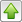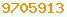# complex : Java Glossary

complex
Complex numbers are pairs of floats or doubles with x being the real part and y being the imaginary part. The imaginary part is though of as being multiplied by the √-1 and added to the real part. They can be thought of geometrically as points on the x/y plane. Complex numbers can also be thought of as points defined by polar coordinates consisting of an angle θ anticlockwise from the x axis and a radius r.

Java has no built-in support for complex numbers. To add or subtract two complex numbers you just add or subtract the corresponding real and imaginary parts.

(a + ib) + (c + id) = (a+b) + i(b+d)

// subtracting two complex numbers:
(a + ib) - (c + id) = (a-b) + i(b-d)

// multiplying two complex numbers:
(a + ib) * (c + id) = (ac - bd) + i(bc + ad)

// dividing two complex numbers:
(a + ib) / (c + id) = ((ac + bd) + i(bc - ad)) / (c² + d²)

// or in practice:
double bottom = 1.d / (c*c + d*d);
double real = (ac + bd) * bottom;
double imaginary = (bc - ad) * bottom;This page is postedon the web at: http://mindprod.com/jgloss/complex.html Optional Replicator mirror of mindprod.com on local hard disk J: J:\mindprod\jgloss\complex.htmlPlease read the feedback from other visitors, or send your own feedback about the site.Contact Roedy. Please feel free to link to this page without explicit permission. Canadian Mind Products IP:[65.110.21.43] Your face IP:[3.238.111.130] Feedback You are visitor number